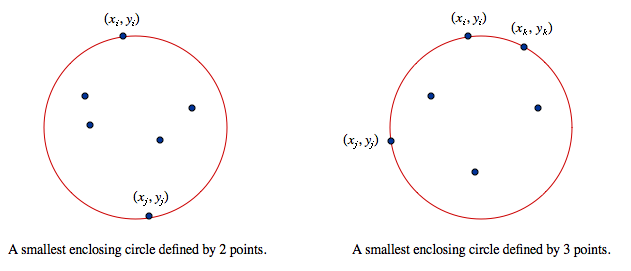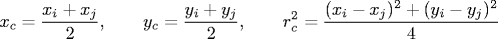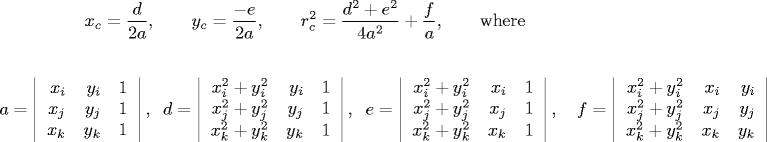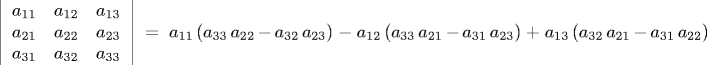## Smallest Enclosing Circle

DRAFT

Given N points in the plane, find the circle of minimum radius that contains all of the points.

Applications. The problem has numerous applications. For example, consider the placement of a new school to service a number of students. If we model the students as points in the plane, the smallest enclosing circle specifies where to build the school: placing the school at the circle's center minimizes the distance between the school and the furthest student. Alternatively, if we view the points as military targets, the minimum enclosing circle specifies where to drop a bomb to destroy the targets, and its radius tell us how much explosive is required.

Structure. The smallest enclosing circle is uniquely defined. Moreover, it is characterized by two or three of the points, which lie on the boundary of the circle.Specifically, the smallest enclosing circle is one of the following:

• A circle whose boundary contains 2 of the N points and whose diameter connects the two points. If the two points are (xi, yi) and (xj, yj), then the circle has center and radius:• A circle whose boundary contains 3 of the N points. If the three points are (xi, yi), (xj, yj), and (xk, yk), then the circle has center and radius:Recall, the determinant of a 3-by-3 matrix is defined as follows:Brute force. Write a program Brute.java that examines all pairs and all triples of points, checks which of the corresponding circles encloses all N points, and outputs the smallest such circle.

An efficient algorithm.

Input format. The data file consists of an integer N, followed by N pairs of real numbers (x, y). The points will all lie in the unit circle: (x2 + y2 ≤ 1).

```% more input5.txt       % more input8.txt
```

Output format. Print the circle on standard output and draw the points and the circle on standard draw. Scale the coordinate system so that coordinates between -1 and +1 fit in the graphics window.

```% java Brute < input5.txt
output

% java Fast < input8.txt
output
```

Analysis. Estimate (using tilde notation) the average-case running time (in seconds) of your two programs as a function of the number of points N (where the points are generated uniformly in the unit circle). Provide empirical evidence to justify your hypotheses.

What is the worst-case running time of your two program as a function of N?

Deliverables. Submit the files: readme.txt, Brute.java, Fast.java, Point.java, and Circle.java. Also submit any other auxiliary files, if any, that your program needs (excluding Std*).

This assignment was developed by Kevin Wayne.